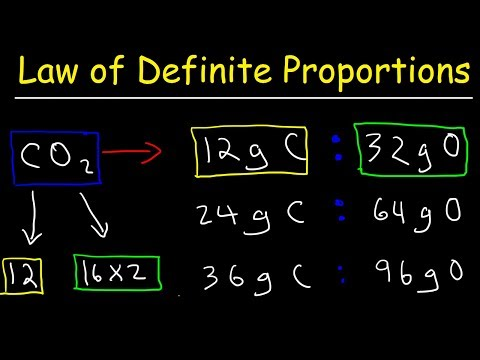Difference Between Law Of Definite Proportions And Law Of Multiple Proportions

## Video: Difference Between Law Of Definite Proportions And Law Of Multiple ProportionsVideo: Law of Definite Proportions Chemistry Practice Problems - Chemical Fundamental Laws 2023, Září

The key difference between law of definite proportions and law of multiple proportions is that the law of definite proportions states the samples of a compound will always contain the same proportion of elements by mass. On the contrary, the law of multiple proportions (sometimes called Dalton’s law) states that if two elements combine to form more than one chemical compound, then the ratios of the masses of the second element that combine with a fixed mass of the first element will be ratios of small whole numbers.

The law of definite proportions and law of multiple proportions are theories used to explain the stoichiometry in chemistry. Stoichiometry is the measure of the relative quantities of reactants and products in a chemical reaction.